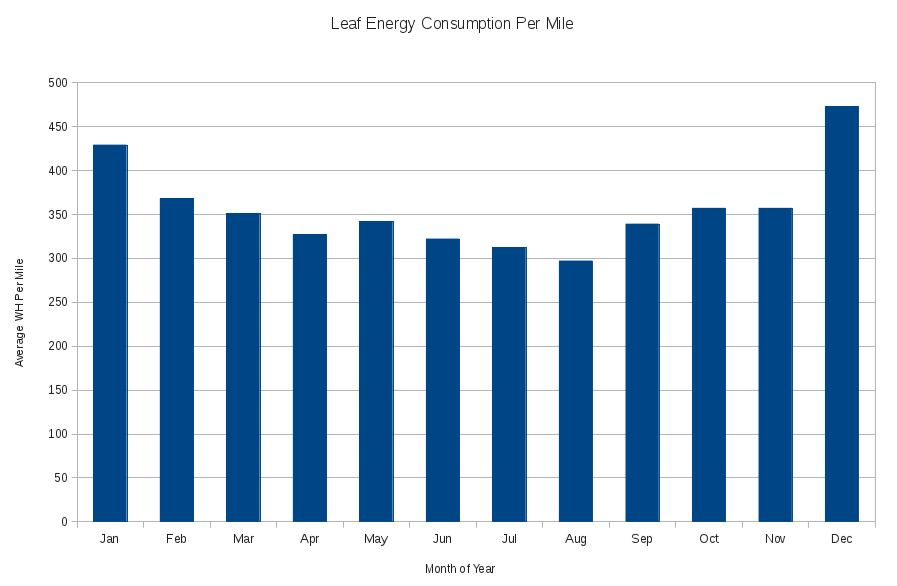# Leaf Energy Consumption By Month

The bar chart below is based on just over 30,000 miles covered by our 2011 Leaf. A major component of the mileage which has gone into compiling the below, is dual carraigeway/motorway commuting. The WH/mile figures below are therefore higher than one might expect if one were driving a higher proportion of distances in 30mph and 40mph zones.

The energy used for the calculation of the below, is the electrical energy which has gone into the car in the last 32 months, as read off an electricity meter installed for the sole purpose of measuring energy used by the car, and so

1. includes the charging inefficiencies (i.e. the car will have used less energy than below)
2. includes all energy used for preheating the car on cold mornings, but
3. excludes the 22 fast charges which the car has received during our ownership (the "22" figure was provided by the Leaf Spy mobile phone app used in conjunction with an ODBII bluetooth interface).As the 2013 Leaf contains a heat pump (rather than crude heater) for cabin heating purposes, it is likely to have less seasonal variation in energy consumptions than our 2011 model (shown above).

I have pasted below the SQL used to compute the average Watt Hours per Mile figures shown in the above graph. The source tables in the below query contain meter reads and car odometer readings for dates. As someone elses SQL is not always the easiest to read, the approach taken by the query below is

1. identify the electricity meter reads (in KWH) and odometer reads (in miles) for the same dates,
2. compute the distance driven for and electricity used between each pair of read dates,
3. spread the distances driven, and electricity used linearly over the dates between each set of readings,
4. aggregate the distances and electricity consumption at month level (ignorring which year the month was in), and
5. divide the aggregated consumption figures by the aggregated mileage figures (and multiply by 1000 to convert KWH to WH) to provide a month average WH per mile figure.

As I have just finished working on the site I have commuted to for the last few years, the graph above uses data from as consistent a set of vehicle usage data as I am likely to have - in order words does not contain data from our revised vehicle usage pattern.

```WITH basic_data AS (
FROM   elec_low
), deltas AS (
odo - LAG(odo) OVER (ORDER BY read_date) AS distance_driven,
FROM   basic_data
), end_dates AS (
FROM   basic_data
), generate_dates AS (
SELECT first_day + (rownum-1) as calc_date, TO_NUMBER(TO_CHAR(first_day + (rownum-1), 'MM')) AS calc_month,
TO_CHAR(first_day + (rownum-1), 'Mon') calc_month_string
FROM   end_dates CROSS
JOIN   all_objects
WHERE  rownum <= last_day - first_day + 1
), day_figures AS (
SELECT calc_date, calc_month, calc_month_string,
FROM   generate_dates a
), month_totals AS (
SELECT calc_month, calc_month_string, SUM(day_distance) AS month_distance, SUM(day_energy) AS month_energy
FROM   day_figures
GROUP BY GROUPING SETS ((calc_month, calc_month_string), ())
)
SELECT NVL(calc_month_string, 'Total') "Month", month_distance, month_energy,
ROUND(1000 * month_energy / month_distance) AS "Avg WH/Mile"
FROM   month_totals
ORDER BY calc_month;

/*
Month        MONTH_DISTANCE MONTH_ENERGY Avg WH/Mile
------------ -------------- ------------ -----------
Jan              1696.86667   728.773333         429
Feb              1693.45903   623.789375         368
Mar               1741.3066    610.90559         351
Apr               2170.8974   710.499134         327
May              2941.33142   1007.36724         342
Jun              3219.32143   1037.30402         322
Jul               3314.0275   1033.53332         312
Aug              3273.33483    972.66766         297
Sep              2701.71707   916.331995         339
Oct              3203.86432   1144.65409         357
Nov              3453.48485   1231.29091         357
Dec              1281.38889   605.583333         473
------------ -------------- ------------ -----------
Total                 30691      10622.7         346
*/
```